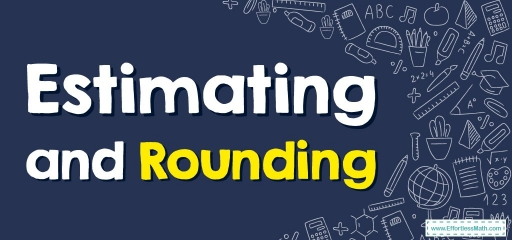# Estimating and Rounding

If you want to know more about how to estimate and round numbers, do not miss our article about it!Estimating and rounding are mathematics tactics utilized for estimating a number. To estimate means you are making a rough guesstimate or a calculation.

If you are rounding, it means you want to simplify a recognized number by scaling it somewhat up or down. So, rounding is a  kind of estimation. Both techniques can aid one in making educated guesses and you can use them in regular life for tasks linked to cash, distance, or time.

## Way to Round Numbers

Rounding involves reducing the number of digits within a number yet ensuring the number stays near the original amount. These potential figures have zeros within the digits on the right (they’re multiples of $$10$$). To round a numeral, you must use the following steps:

Step one: Locate the “round off” number. The round-off number is the place value of the figure you want to round up or down to. For instance, if you wish to round a figure to the closest ten, the round-off number is the one located in the tens’ place. If you wish to round it to the closest hundred, the round-off number is the number seen in the hundreds’ position.

Step two: Look to the number on the right of the rounding number. If it is $$5$$ or more, take the rounding number up to one digit. If this is lower than $$5$$, take it down one digit.

Step three: Change every number on the right of the rounded-off digit to $$0$$s.

Note: Whenever a figure is halfway in between the $$2$$ likely figures, round it up to the larger figure.

### Estimating and Rounding– Example 1:

Estimate the sum by rounding a number to the nearest ten.

$$32+87=$$

Solution:

First-round the numbers to the nearest ten. Look to the number on the right of the rounding number. The number on the right of $$32$$ is $$2$$. This is lower than $$5$$, so, round down. It will be $$30$$.

The number on the right of $$87$$ is $$7$$. This is more than $$5$$, so, round it up. It will be $$90$$.

Now add the two numbers together $$= 30+90=120$$

### Estimating and Rounding– Example 2:

Estimate the product by rounding a number to the nearest ten.

$$11 × 26=$$

Solution:

First-round the numbers to the nearest ten. Look to the number on the right of the rounding number. The number on the right of $$11$$ is $$1$$. This is lower than $$5$$, so, round down. It will be $$10$$.

The number on the right of $$26$$ is $$6$$. This is more than $$5$$, so, round it up. It will be $$30$$.

Now multiply the two numbers together $$= 10 × 20=200$$

## Exercises for Estimating and Rounding

Estimate the sum or product by rounding each number to the nearest ten.

1. $$\color{blue}{35+51=}$$
2. $$\color{blue}{23+44=}$$
3. $$\color{blue}{13×19=}$$
4. $$\color{blue}{67×34=}$$
1. $$\color{blue}{90}$$
2. $$\color{blue}{60}$$
3. $$\color{blue}{200}$$
4. $$\color{blue}{2,100}$$

### What people say about "Estimating and Rounding - Effortless Math: We Help Students Learn to LOVE Mathematics"?

No one replied yet.

X
30% OFF

Limited time only!

Save Over 30%

SAVE $5 It was$16.99 now it is \$11.99